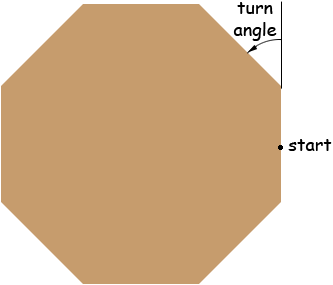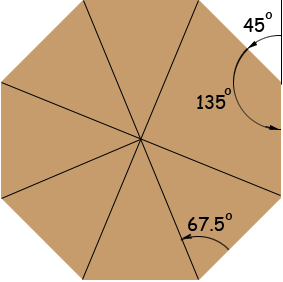SEARCH HOMEMath Central Quandaries & QueriesSubject: octagon Name: Verner Who are you: Other I am building a octagon birdhouse,what degree would I cut each side of each piece of wood to assemble the birdhouse?Hi Verner,

Start in the middle of one side of the octagon and walk around the perimeter in a counterclockwise direction. Each time you reach a corner you need to turn a certain angle to remain on the perimeter. The angle is the same at each corner.When you are back at the start position you have turned a total of 360 degrees. You did this through 8 turns of the same amount so each turn was 360/8 = 45 degrees. Thus if you are making a one piece octagonal base for your birdhouse then you need to cut 45 degree angles.

Since the turn angle at each vertex measures 45 degrees the interior angle at each vertex measures 180 - 45 = 135 degrees.Thus if yo join a vertex to the centre of the octagon you form an angle measuring 135/2 = 67 1/2 degrees.

If you have a digital camera, when you complete the birdhouse send us a photo,
PennyMath Central is supported by the University of Regina and The Pacific Institute for the Mathematical Sciences.